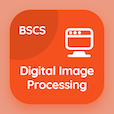Engineering Online Courses

Electromagnetic Theory Quizzes

Electromagnetic Theory Quiz PDF - Complete

# Finite Difference Time Domain Popularity Multiple Choice Questions p. 17

Study Finite Difference Time Domain Popularity multiple choice questions and answers, finite difference time domain popularity quiz answers PDF 17 to study Electromagnetic Theory course online. Metamaterials MCQ trivia questions, Finite Difference Time Domain Popularity Multiple Choice Questions (MCQ) for online college degrees. "Finite Difference Time Domain Popularity MCQ" PDF eBook: curl operator, dielectric permittivity, chiral metamaterials, lorentz force, finite difference time domain popularity test prep for best online colleges.

"FDTD avoids the difficulties with linear algebra that limit the size of" MCQ PDF: frequency domain integral equation, frequency domain differential equation, time domain differential equation, and time domain integral equation for job placement test. Learn metamaterials questions and answers to improve problem solving skills for associate degrees in engineering.

## Finite Difference Time Domain Popularity Questions and Answers MCQs

MCQ: FDTD avoids the difficulties with linear algebra that limit the size of

frequency domain differential equation
frequency domain integral equation
time domain differential equation
time domain integral equation

MCQ: Law which governs the interaction of the electromagnetic field with charged matter.

Gauss's Law
Ampere's Law
Lorentz force Law

MCQ: Swiss roll structure of chiral metamaterial is wound in a

straight manner
helical manner
triangular manner
round manner

MCQ: Xe=

P/(∊E)
P∊E
(∊E)/P
P/∊

MCQ: Small vector which direction defines field vector is

displacement vector
unit vector
curl vector

### More Quizzes from Electromagnetic Theory Course